Which strategy maths cards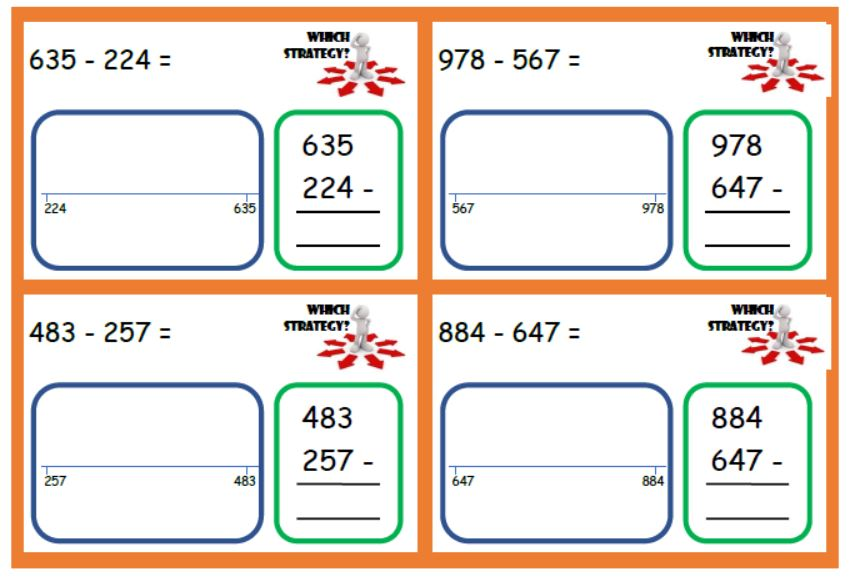Which strategy maths cards

Free download to help children see that they can solve maths problems in a variety...

Divide by 12 Fortune Teller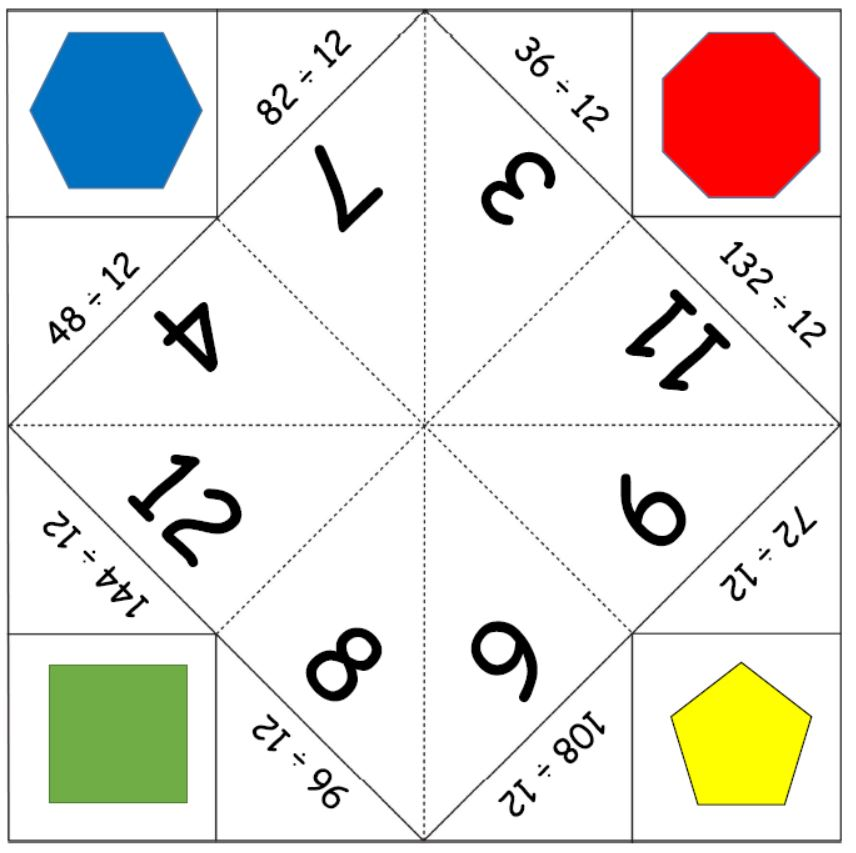Divide by 12 Fortune Teller

A fun way to practise times tables- a free printable for the divide by 12 tables...

Divide by 9 Fortune TellerDivide by 9 Fortune Teller

A fun way to practise times tables- a free printable for the divide by 9 tables to...

Divide by 8 Fortune Teller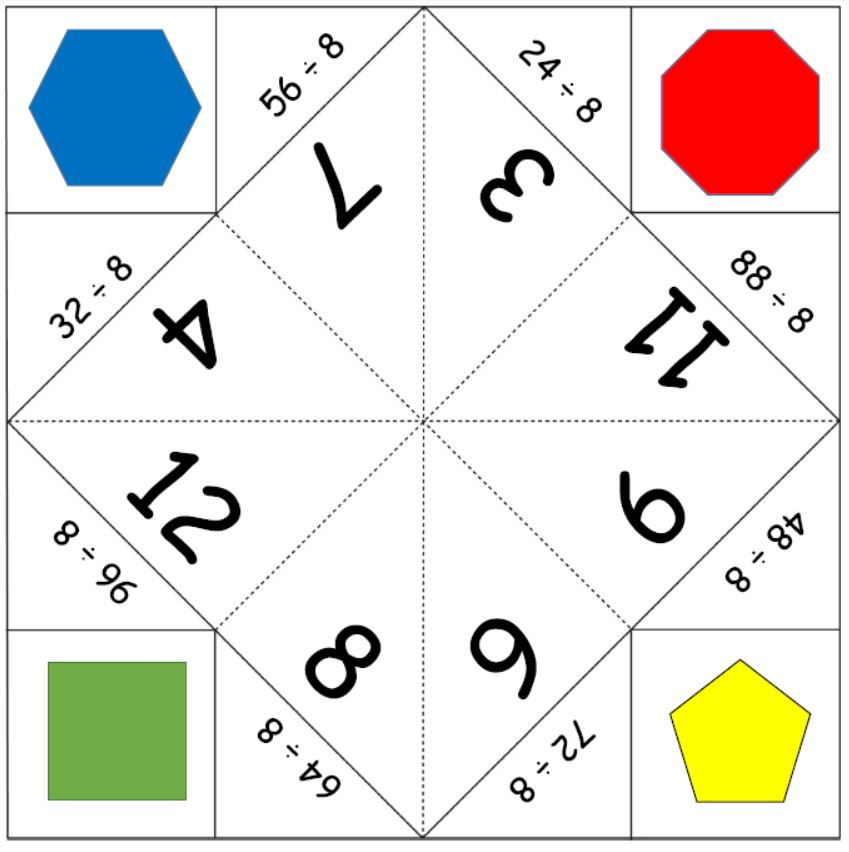Divide by 8 Fortune Teller

A fun way to practise times tables- a free printable for the divide by 8 tables to...

Divide by 7 Fortune Teller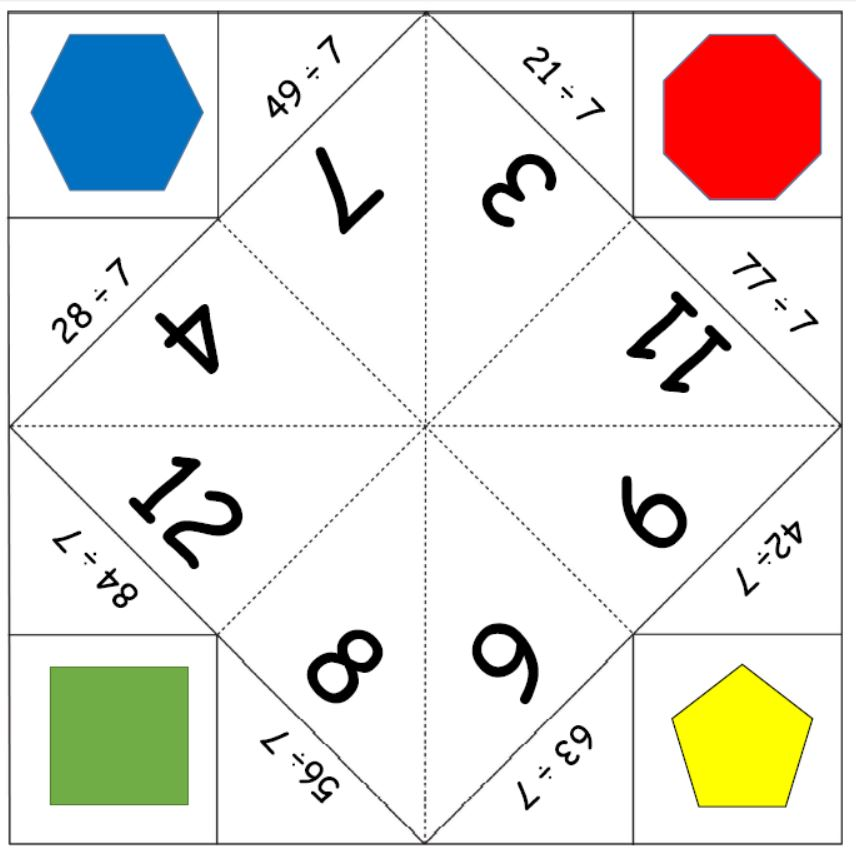Divide by 7 Fortune Teller

A fun way to practise times tables- a free printable for the divide by 7 tables to...

Divide by 6 Fortune Teller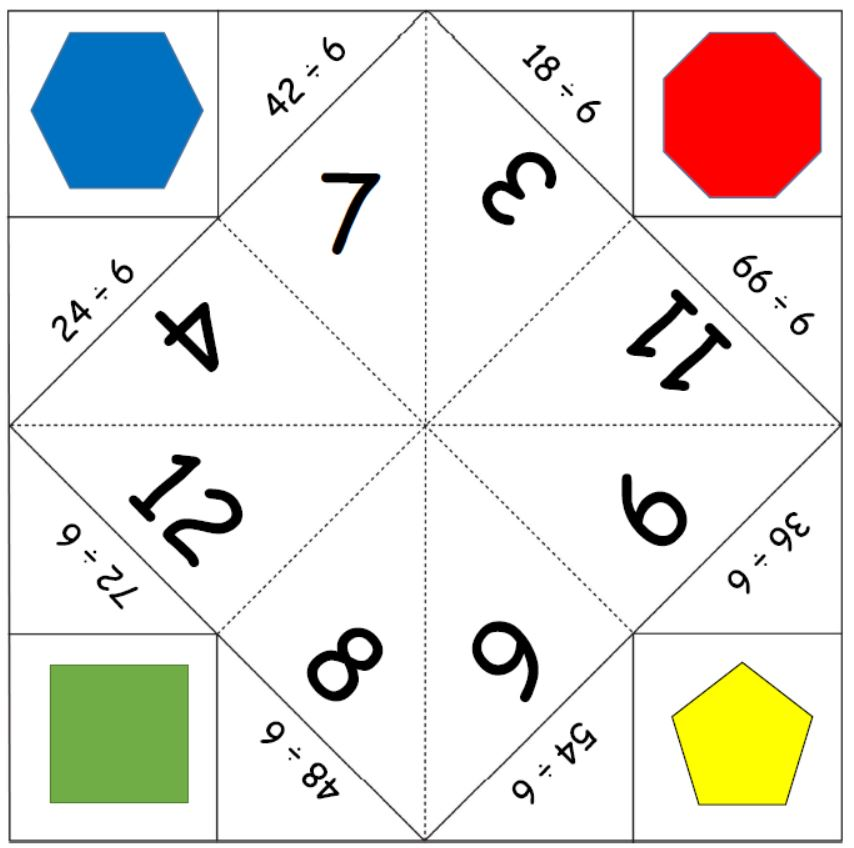Divide by 6 Fortune Teller

A fun way to practise times tables- a free printable for the divide by 6 tables to...

Divide by 5 Fortune Teller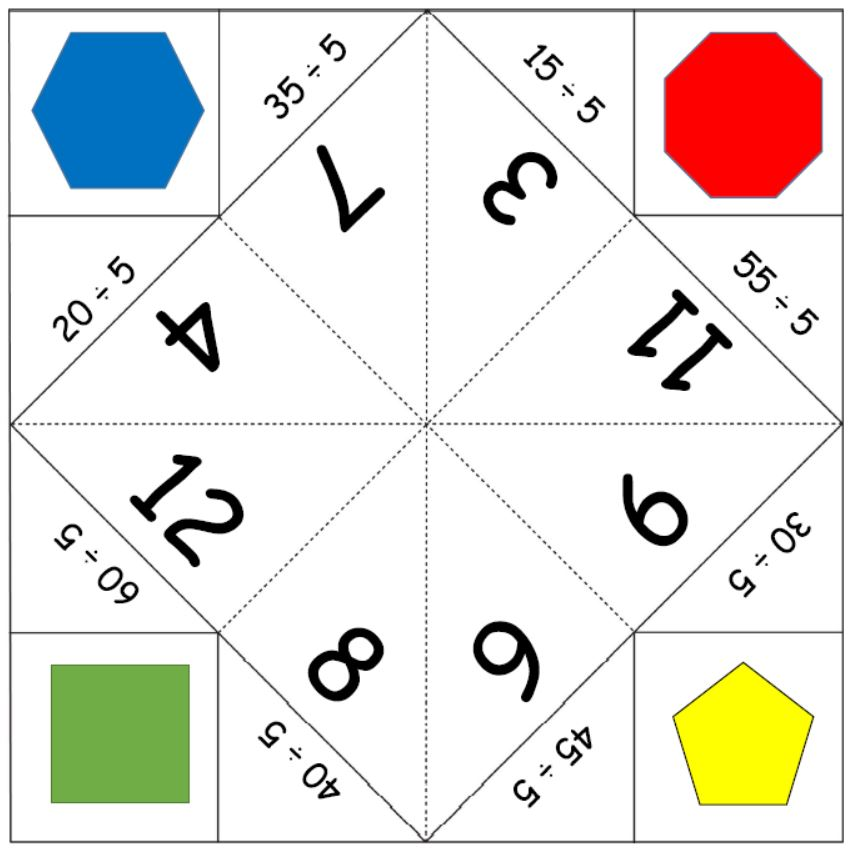Divide by 5 Fortune Teller

A fun way to practise times tables- a free printable for the divide by 5 tables to...

Stage 2 division sum cardsStage 2 division sum cards

Free printable - 3 cards for practising division - Stage 2 Division sum cards. ...

Division with remainder sheet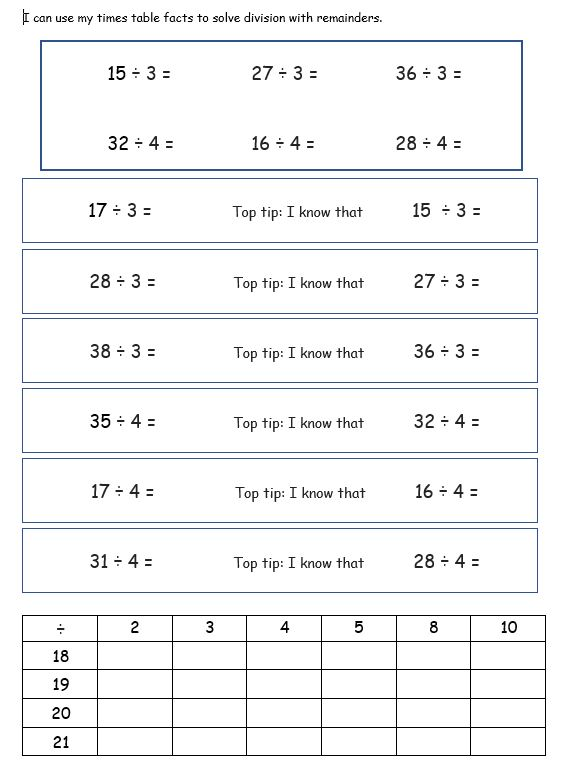Division with remainder sheet

Free printable to help with learning about division with remainders - based on knowing...